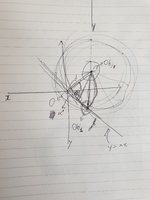# Get position of object when rotated alpha° based on mid point O

#### DankRed2

##### New member
I need to get position of a object (can be anything) when rotated alpha° based on mid point O.

I tried to solve this by using a feature of circle and line's meeting point- but there were several issues regarding, but first I will tell you the conditions.

- I know the value alpha
- I know where exactly object is before I rotate it = know the distance between O and not-moved object's middle point.
- Mid point O is (0, 0)
- You can make a lot of triangles and circles to solve this, but I want simplest way to solve this- even its quick-and-dirty way.
- Distance between object and O is ALWAYS SAME.

Now the issues,

- I tried to solved this by using x=0 or y=0 between moved position and feature of circle and a line's meeting point (circle is made of a line between moved and not-moved object's middle point, line is between O and moved object's middle point). Has a limitation because when moving to 351 to 348 didn't have x=0 or y=0 at the middle (does not have to be exact) of it. Any other way than drawing y=-4x or such things like this?
- As I mentioned "meeting point" above, sometimes it was not a single point. So I need to know condition to programmatically define to choose a single point. Just tell me how I can logically tell which point is the point I actually want.

#### Dr.Peterson

##### Elite Member
This is very hard to follow. Can you show a picture? Do you know any trigonometry?

•DankRed2

#### DankRed2

##### New member
This is very hard to follow. Can you show a picture? Do you know any trigonometry?I tried to visualize this, however it does not always look like this because this can be any* shape based on this' structure. Hopefully it is visable enough.. (I will update this picture as soon as I get home.*)

What I know is location of Obj0, and distance between it and O*, and alpha. I need to know Obj1's exact location.

As you see y=mx line* overlaps 2 part of point circle and in these two, location of Obj1 is what I want.*

Also, I only know the very basic of trigonometry.. I can't use any of cos rule at all.

Edit: fixed typo at *

#### HallsofIvy

##### Elite Member
What is confusing (to me) is that you say "based on mid point O" but then say "I ... know the distance between O and not-moved object's middle point."

So "mid-point O" is NOT the "object's middle point"? Then what is it the mid point of?

I think you mean simply rotating an object around a given "center of rotation", not a "mid point".

To do that, use the fact that the a single point (x, y), rotated around (0, 0) through angle $$\displaystyle \alpha$$ will move to point $$\displaystyle (x+ r \cos(\alpha), y+ r \sin(\alpha))$$ where r is the distance from (0, 0) to (x, y), $$\displaystyle r= \sqrt{x^2+ y^2}$$. If the center of rotation is $$\displaystyle (a, b)$$, not (0,0), first translate to the origin, then rotate, then translate back: $$\displaystyle (x_0, y_0)\to (x_1, y_1)= (x_0- a, y_0- b)\to (x_1+ r cos(\alpha), y_1+ r sin(\alpha))=(x_2, y_2)\to (x_2+ a, y_2+ b)$$.

•DankRed2

#### DankRed2

##### New member
What is confusing (to me) is that you say "based on mid point O" but then say "I ... know the distance between O and not-moved object's middle point."

So "mid-point O" is NOT the "object's middle point"? Then what is it the mid point of?

I think you mean simply rotating an object around a given "center of rotation", not a "mid point".

To do that, use the fact that the a single point (x, y), rotated around (0, 0) through angle $$\displaystyle \alpha$$ will move to point $$\displaystyle (x+ r \cos(\alpha), y+ r \sin(\alpha))$$ where r is the distance from (0, 0) to (x, y), $$\displaystyle r= \sqrt{x^2+ y^2}$$. If the center of rotation is $$\displaystyle (a, b)$$, not (0,0), first translate to the origin, then rotate, then translate back: $$\displaystyle (x_0, y_0)\to (x_1, y_1)= (x_0- a, y_0- b)\to (x_1+ r cos(\alpha), y_1+ r sin(\alpha))=(x_2, y_2)\to (x_2+ a, y_2+ b)$$.
Solved the issue, yes you are right. I meant by simply rotating object around (0, 0). Now I see a lot of errors in my question, I will try to improve this question later (when my English skill gets somewhat decent) for anyone having a same question on the internet and looking for an answer! Appreciate your volunteering.